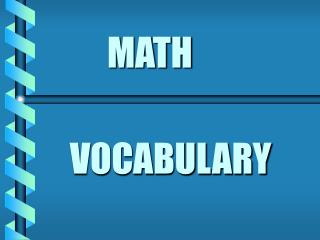DownloadDownload PresentationMATH

# MATH

Télécharger la présentation## MATH

- - - - - - - - - - - - - - - - - - - - - - - - - - - E N D - - - - - - - - - - - - - - - - - - - - - - - - - - -
##### Presentation Transcript

1. MATH VOCABULARY

2. VOCABULARY SUM TOTAL DIFFERENCEMINUS PRODUCTQUOTIENT INCREASEDECREASE VARIABLEEVALUATEESTIMATEEQUATION MULTIPLE FACTOR EXPRESSIONSUBSTITUTE

4. TOTAL The whole amount. Sum.

5. DIFFERENCE The answer to a subtraction problem.

6. MINUS To make smaller by subtracting.

7. PRODUCT The answer to a multiplication problem.

8. QUOTIENT The answer to a division problem.

9. INCREASE To become larger.

10. Decrease To become smaller.

11. Variable A letter that represents a number.

12. EVALUATE To find the value of.

13. ESTIMATE To make a careful guess about the size or amount.

14. EQUATION A number sentence.

15. MULTIPLE The product of that number and another whole number.

16. FACTOR A number that divides evenly into another number.

17. EXPRESSION A math statement written using variables and/or numbers.

18. SUBSTITUTE To use in place of something.